How to Highlight a row on the basis of Cell

In this article, we will learn How to Highlight a row on the basis of Cell

Earlier we learned how to highlight entire if any cell in row contains specific text. In this article, we will learn how to highlight row if cell in a contains a specific value. Not only text, if any criteria is met, we will highlight the row using conditional formating.

Generic Formula in Conditional Formating

 = (cell with relative row = criteria)

Cell with relative row: this is the a cell reference where column is absolute and row is relative. For example \$A1. Learn in detail about referencing in excel here.

Criteria: It is the criteria that you want to match and highlight row. It can be a text a value or logical test.

Let’s see an example to make things clear.

Example: Highlight Row Based on Cell Value of Rates

Here I have some data of fruits. I just want to highlight entire row if fruit rate is greater then 100.

Select first row of table (A2:C2).

1. Go to conditional formatting and click on new rule. You can use sequential shortcut ALT>H>L>N.
2. Select “use a formula to determine which cell to format”.
3. Write this formula.
 =\$C2>100

It is necessary to select the entire row before doing conditional formatting.

1. Click on format and choose yellow in fill. Hit ok.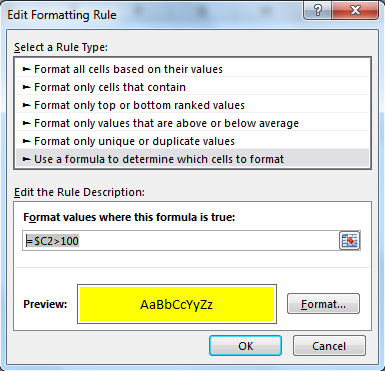Copy this range and paste special the format to entire table.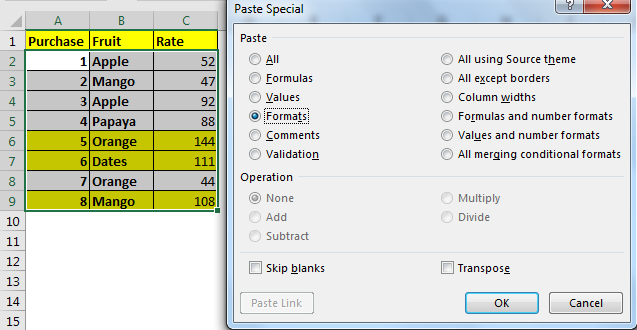Now each row will be highlighted whose rate is greater than 100. Here I have hardcoded the criteria, but you can give cell reference too.

How it works?

Well, it is quite simple.  We simply check if value in \$C2 is greater than 100 or not. If it is, we tell excel to highlight the entire row.

Note that only Column C is frozen using \$ and row are left relative. It is because we want to change the lookup row when we copy the conditional formatting and while column stands absolute. In the end we get highlighted rows that contains value greater than 100. Learn about referencing in detail here.

Alternate method

Example :

All of these might be confusing to understand. Let's understand this formula by running it on an example. Here we have data with estimated population for each region.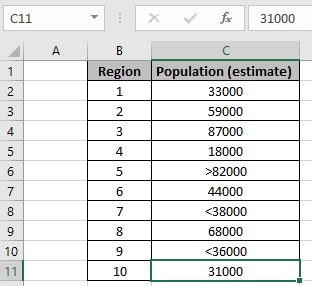Here as you can see the data have values (numbers with operator) which need to be considered too. We need to highlight cells which have a population less than 50000. So we use the formula stated below with Explanation under Conditional formatting formula box.

Go to Home -> Conditional formatting -> New Rule

A dialog box appears in front select Use a formula to determine which cells to format -> input the below formula under the Format values where this formula is True:

Use the formula for matching value less than 50000.

 =IF( ISNUMBER (C2) ,C2 < \$G\$3 , IF( LEFT (C2) = "<" ,(MID (C2 , 2 , LEN (C2 ) + 0) < \$G\$3 ) )

Explanation:

1. First IF function checks if the value is a number or not using the ISNUMBER function.
2. If the value is a number, then the number is directly matched with criteria and returns True or False.
3. But if the value is the number with operator, then first we extract the operator and match it with less than ("<") operator, then the remaining is the number to match with criteria and returns True or False.

MID (C2 , 2 , LEN (C2 ) + 0), here MID function returns the value ignoring operator(char) and returns the value in number format using (+0).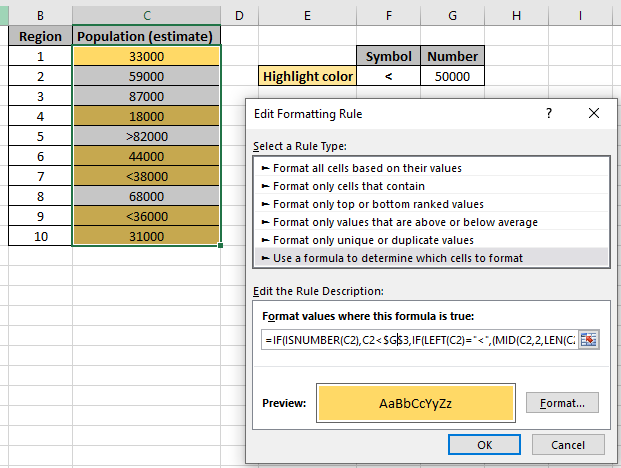As you can see the formula, the yellow background is the highlighted and the required data. You can check the formula in a sample cell to verify whether the value returns True or False. Now i will add one more rule to highlight the population which is greater than 80000. Create new rule in conditional formatting and use the below formula to highlight the numbers with green background.

Use the formula for matching value less than 50000.

 =IF( ISNUMBER (C2) ,C2 > \$G\$4 , IF( LEFT (C2) = ">" ,(MID (C2 , 2 , LEN (C2 ) + 0) > \$G\$4 ) )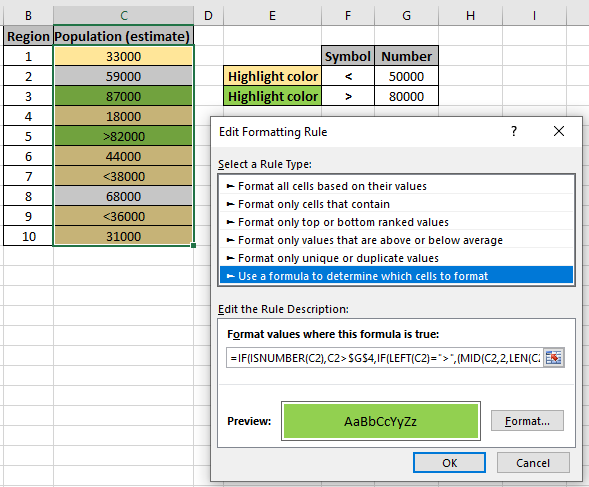As you can see, Conditional formatting does all the highlighting for you.

Here are all the observational notes regarding using the formula.

Notes:

1. Conditional formatting allows you to apply the formatting basis on the cell values such as colours, icons and data bars.
2. The formula works for text and numbers both.

Hope you understood How to Highlight a row on the basis of Cell. Explore more articles on Excel highlighting cells with formulas here. If you liked our blogs, share it with your friends on Facebook. And also you can follow us on Twitter and Facebook. We would love to hear from you, do let us know how we can improve, complement or innovate our work and make it better for you. Write to us at info@exceltip.com.

Related Articles:

Find the partial match number from data in Excel : find the substring matching cell values using the formula in Excel.

How to Highlight cells that contain specific text in Excel : Highlight cells based on the formula to find the specific text value within the cell in Excel.

Conditional formatting based on another cell value in Excel : format cells in Excel based on the condition of another cell using some criteria in Excel.

IF function and Conditional formatting in Excel : How to use IF condition in conditional formatting with formula in Excel.

Perform Conditional Formatting with formula 2016 : Learn all default features of Conditional formatting in Excel.

Conditional Formatting using VBA in Microsoft Excel : Highlight cells in the VBA based on the code in Excel.

Popular Articles :

50 Excel Shortcut to Increase Your Productivity : Get faster at your task. These 50 shortcuts will make you work even faster on Excel.

How to use the VLOOKUP Function in Excel : This is one of the most used and popular functions of excel that is used to lookup value from different ranges and sheets.

How to use the COUNTIF function in Excel : Count values with conditions using this amazing function. You don't need to filter your data to count specific values. Countif function is essential to prepare your dashboard.

How to use the SUMIF Function in Excel : This is another dashboard essential function. This helps you sum up values on specific conditions.

1.You've got the range wrong in the rule formula. It doesn't cover rows 8-14.

It should be =\$AA8=MAX(\$AA\$8:\$AA\$46)

•Hi Dave,

We have corrected the sheet.

But thanks for bringing in our notice.

Best regards,
Team Excel Forum & Excel Tip

2.It appears row 14 has a higher DGPA and should be highlighted instead of row 21.

•Hi Ron,

Thanks for your feedback. We have checked it and observed that due to incorrect range, it was highlighting the highest DGPA from AA15:AA56.

Now we have updated the sheet.

Thanks,
Team Excel Forum & Excel Tip

3.I believe the statement "Select the range DGPA AA8:AA46" should be "Select the range DGPA A8:AA46" (A8 vs AA8) to get the entire row to highlight. The actual example in the workbook is correct.

•Hi Ron,

Thanks for bringing in our notice.

The formula is used in conditional formatting to highlight the row basis on highest DGPA from the column range AA8:AA46.

Team Excel Tip & Excel Forum

Terms and Conditions of use

The applications/code on this site are distributed as is and without warranties or liability. In no event shall the owner of the copyrights, or the authors of the applications/code be liable for any loss of profit, any problems or any damage resulting from the use or evaluation of the applications/code.